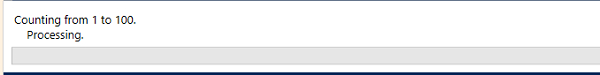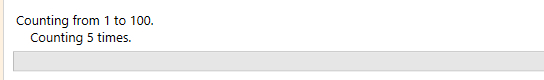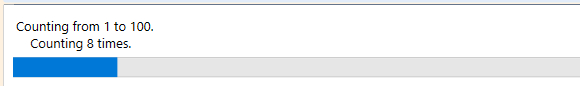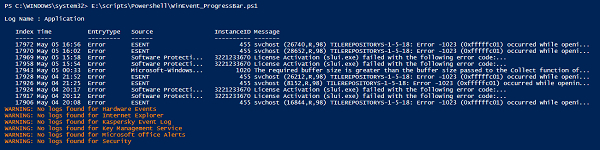# How to write Progress Bar in PowerShell?

When we write a script in PowerShell, users who execute it, expect to see the progress of the script instead of waiting idle and looking at the blank cursor while the script is executing the background task. One way to achieve is to use the Verbose parameter to see the progress but it doesn’t show the graphical progress. To see the graphical progress, you can use Write-Progress cmdlet supported by PowerShell.

Write-Progress cmdlet mainly depends on 3 parameters.

• Activity − Title of the progress bar or you can mention the activity name that is being performed.

• Status − Subtitle of the Progress bar. This would be the second line after activity.

• Percentage Completed − Percentage completed in terms of progress. This should be the integer number.

Check the below example with just the activity parameter and run the code.

for($i=0;$i -le 100; $i++){ Write-Progress -Activity "Counting from 1 to 100" sleep 1 } ## OutputIn the above output, you can see that the “Counting from 1 to 100” is the header, and “Progressing” is the default status that we haven’t modified yet. Here you can see there is not progress shown as we haven’t used the Percentage parameter. Now we will modify the Status of the progress bar in the same example. ## Example for($i=0;$i -le 100;$i++){
Write-Progress -Activity "Counting from 1 to 100 " -Status "Counting $i times"$i
sleep 1
}

## OutputAs you can see the above output with the new subtitle but still we don’t see the percentage completed and for that, you need to use the PercentComplete parameter.

$tcount = 100 for($i=0;$i -le$tcount; $i++){$pcomplete = ($i /$tcount) * 100
Write-Progress -Activity "Counting from 1 to 100" -Status "Counting $i times" -PercentComplete$pcomplete
$i sleep 1 } When you see the output of the above code, you will see the progress bar working.We now see another example which will help us to get more ideas about the progress bar. In the below example we have used Windows Event command to retrieve the first 10 error logs from each windows event like System, Application, security, etc and while the retrieval process takes a little time, we will use the progress bar to display the growth of the script output. ## Example $Winlogs = (Get-Eventlog -List).LogDisplayName
foreach($log in$Winlogs){
try{
$eventlog = Get-EventLog -LogName$log -EntryType Error -ErrorAction Stop| Select -First 10
if($eventlog){ Write-Output "nLog Name :$log"
for($i=0;$i -le $eventlog.count;$i++){
$perct = ($i / $eventlog.count) * 100 Write-Progress -Activity "Windows Logs Progress" -Status "Displaying Log :$log" -                 PercentComplete $perct$eventlog[$i] Sleep 1 } } } catch [Exception]{ Write-Warning "No logs found for$log"
}
}`

## Output